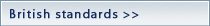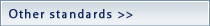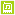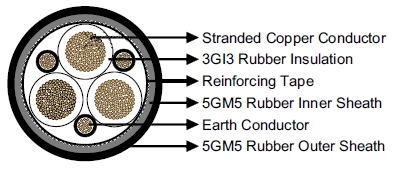Mining CableType WType GType G-GCmoreMining Cable

NTSCGEWOEU Flexible Medium-Voltage Trailing Cable

Applications

These cables are used as power supply or connection cables for large material handling machines, e.g.

excavators in open-cast mines subject to extremely high mechanical stresses. Particularly suitable for

applications in which abrasion and chaffing stresses are to be expected in trailing operation.

Standards

VDE 0250 Part 813

ConstructionConductors: Flexible stranded tinned copper conductor, class 5 according to DIN VDE 0295.

Inner Conductor Layer: Semiconductive layer.

Insulation: Rubbertype 3GI3.

Outer Conductor Layer:Semiconductive layer.

Earth Conductor: Split into three in theouter interstices or Individual concentric distributed over core insulating

coverings (coding…../3E).

Reinforcing Tape: Extremely tear-resistant reinforcing tape.

InnerSheath: Rubber type 5GM5, abrasion and tear resistant, oil and ozone resistant.

Outer Sheath: Rubber type 5GM5, abrasion and tear resistant, oil and ozone resistant, inseparably bonded with

inner sheath.

Dimensions and Weight

1.8/3kV

 Number of Cores×Nominal Cross Section Minimium Overall Diameter Maximum Overall Diameter Nominal Weight No.×mm² mm mm kg/km 3×25+3×25/3 38.5 41.5 2470 3×35+3×25/3 42.9 45.9 3080 3×50+3×25/3 46.1 49.1 3750 3×70+3×35/3 49.7 53.7 4690 3×95+3×50/3 57.4 61.4 6210 3×120+3×70/3 61.2 65.2 7430 3×150+3×70/3 66.7 70.7 8900 3×185+3×95/3 70.6 74.6 10330 3×25+2×25/2+1×10ST 40.3 44.3 2470 3×35+2×25/2+1×10ST 42.9 46.9 3080 3×50+2×25/2+1×10ST 46.8 50.8 3750 3×70+2×35/2+1×10ST 51.5 55.5 4690 3×95+2×50/2+1×10ST 57.4 62.4 6210 3×120+2×70/2+1×10ST 63.6 68.6 7430 3×150+2×70/2+1×10ST 67.2 72.2 8900 3×185+2×95/2+1×10ST 70.2 75.2 10330
3.6/6kV
 Number of Cores×Nominal Cross Section Minimium Overall Diameter Maximum Overall Diameter Nominal Weight No.×mm² mm mm kg/km 3×25+3×25/3 44.6 47.6 3080 3×35+3×25/3 47.6 50.6 3590 3×50+3×25/3 52.4 56.4 4520 3×70+3×35/3 56.3 60.3 5520 3×95+3×50/3 59.9 63.9 6580 3×120+3×70/3 65.6 69.6 8110 3×150+3×70/3 69.3 73.3 9320 3×185+3×95/3 73.2 77.2 10780 3×25+2×25/2+1×10ST 45.0 49.0 3200 3×35+2×25/2+1×10ST 47.6 51.6 3680 3×50+2×25/2+1×10ST 53.0 57.0 4640 3×70+2×35/2+1×10ST 56.2 60.2 5550 3×95+2×50/2+1×10ST 61.8 66.8 6650 3×120+2×70/2+1×10ST 66.1 71.1 8160 3×150+2×70/2+1×10ST 69.8 74.8 9340 3×185+2×95/2+1×10ST 74.6 79.6 10890
6/10 kV
 Number of Cores×Nominal Cross Section Minimium Overall Diameter Maximum Overall Diameter Nominal Weight No.×mm² mm mm kg/km 3×25+3×25/3 46.4 49.4 3270 3×35+3×25/3 49.1 53.1 3800 3×50+3×25/3 54.1 58.1 4750 3×70+3×35/3 58.0 62.0 5750 3×95+3×50/3 61.7 65.7 6830 3×120+3×70/3 67.4 71.4 8380 3×150+3×70/3 71.0 75.0 9620 3×185+3×95/3 76.7 80.7 11430 3×25+2×25/2+1×10ST 46.8 50.8 3410 3×35+2×25/2+1×10ST 50.9 54.9 3890 3×50+2×25/2+1×10ST 54.5 58.9 4860 3×70+2×35/2+1×10ST 58.0 62.0 5780 3×95+2×50/2+1×10ST 63.5 68.5 6920 3×120+2×70/2+1×10ST 67.8 72.8 8450 3×150+2×70/2+1×10ST 71.5 76.5 9620 3×185+2×95/2+1×10ST 76.3 81.3 10980
8.7/15 kV
 Number of Cores×Nominal Cross Section Minimium Overall Diameter Maximum Overall Diameter Nominal Weight No.×mm² mm mm kg/km 3×25+3×25/3 52.6 56.6 4040 3×35+3×25/3 55.6 59.6 4630 3×50+3×25/3 58.9 62.9 5370 3×70+3×35/3 64.5 68.5 6720 3×95+3×50/3 68.2 72.2 7850 3×120+3×70/3 72.1 76.1 9130 3×150+3×70/3 77.6 81.6 10750 3×185+3×95/3 81.5 85.5 12290 3×25+2×25/2+1×10ST 53.0 57.0 4130 3×35+2×25/2+1×10ST 55.6 59.6 4740 3×50+2×25/2+1×10ST 59.3 63.3 5470 3×70+2×35/2+1×10ST 64.6 68.6 6820 3×95+2×50/2+1×10ST 68.3 73.3 7950 3×120+2×70/2+1×10ST 74.4 79.4 9240 3×150+2×70/2+1×10ST 78.1 83.1 10860 3×185+2×95/2+1×10ST 81.1 86.1 12400
12/20 kV
 Number of Cores×Nominal Cross Section Minimium Overall Diameter Maximum Overall Diameter Nominal Weight No.×mm² mm mm kg/km 3×25+3×25/3 56.9 60.9 4620 3×35+3×25/3 59.9 63.9 5220 3×50+3×25/3 65.0 69.0 6300 3×70+3×35/3 68.9 72.9 7410 3×95+3×50/3 72.5 76.5 8560 3×120+3×70/3 78.2 82.2 10260 3×150+3×70/3 81.9 85.9 11570 3×185+3×95/3 87.4 92.4 13530 3×25+2×25/2+1×10ST 57.3 61.3 4770 3×35+2×25/2+1×10ST 59.9 63.9 5340 3×50+2×25/2+1×10ST 65.4 69.4 6460 3×70+2×35/2+1×10ST 68.8 72.8 7450 3×95+2×50/2+1×10ST 74.4 79.4 8680 3×120+2×70/2+1×10ST 78.7 83.7 10370 3×150+2×70/2+1×10ST 82.2 87.2 11650 3×185+2×95/2+1×10ST 87.0 92.0 13090
14/25kV
 Number of Cores×Nominal Cross Section Minimium Overall Diameter Maximum Overall Diameter Nominal Weight No.×mm² mm mm kg/km 3×25+2×25/2+1×10ST 64.7 68.7 5940 3×35+2×25/2+1×10ST 67.3 71.3 6470 3×50+2×25/2+1×10ST 71.0 75.0 7300 3×70+2×35/2+1×10ST 75.2 80.2 8800 3×95+2×50/2+1×10ST 80.0 85.0 10050 3×120+2×70/2+1×10ST 85.9 90.9 11470 3×150+2×70/2+1×10ST 89.6 94.6 13210 3×185+2×95/2+1×10ST 92.6 97.6 14860
18/30kV
 Number of Cores×Nominal Cross Section Minimium Overall Diameter Maximum Overall Diameter Nominal Weight No.×mm² mm mm kg/km 3×25+3×25/3 69.5 73.5 6680 3×35+3×25/3 72.5 76.5 7380 3×50+3×25/3 77.6 81.6 8460 3×70+3×35/3 81.5 85.5 9690 3×95+3×50/3 84.9 89.9 10960 3×120+3×70/3 90.6 95.6 12830 3×150+3×70/3 94.3 99.3 14250 3×185+3×95/3 100.0 105.0 16390 3×25+2×25/2+1×10ST 69.9 73.9 7100 3×35+2×25/2+1×10ST 72.6 76.6 7540 3×50+2×25/2+1×10ST 78.0 82.0 8680 3×70+2×35/2+1×10ST 80.4 85.4 9760 3×95+2×50/2+1×10ST 86.8 91.8 11100 3×120+2×70/2+1×10ST 91.1 96.1 12980 3×150+2×70/2+1×10ST 94.8 99.8 14350 3×185+2×95/2+1×10ST 99.6 104.6 15870